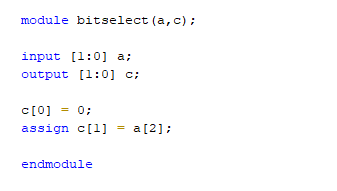## Import Verilog Code and Generate Simulink Model

To import synthesizable HDL code into the Simulink® modeling environment, use HDL import. HDL import parses the input HDL file and generates a Simulink model. The model is a block diagram environment that visually represents the HDL code in terms of functionality and behavior. By importing the HDL code into Simulink, you can verify the functionality of the HDL code by compiling and running simulation on the model in a model-based simulation environment. You can also debug internal signals by logging the signals as test points.

### HDL Import

Roundtrip code generation with HDL import is not recommended. Do not use HDL import to import the HDL code that was previously generated from a Simulink model by using the HDL Coder™ software. The Simulink model that you create is typically at a higher abstraction level. The model generated by HDL import might be at a lower abstraction level. The HDL code you generate from this model might not be usable for production code.

To generate production HDL code, develop your algorithm by using Simulink blocks, MATLAB® code, or Stateflow® charts. Then, use HDL Coder to generate code.

### HDL Import Requirements

To generate a Simulink model, make sure that the HDL file you import:

• Is free of syntax errors.

• Is synthesizable.

• Uses supported Verilog® constructs for the import.

### Import HDL Code

To import the HDL code, in the MATLAB Command Window, run the `importhdl` function. The function parses the HDL input file that you specified and generates the corresponding Simulink model, and provides a link to open the model.

For example, consider a Verilog code of a comparator,

```// File Name: comparator.v // This module implements a simple comparator module `define value 12 module comparator (clk, rst, a, b); input clk, rst; input [1:0] a; output reg [1:0] b; parameter d = 2'b11; always@(posedge clk) begin if (rst) b <= 0; else if (a < `value) b <= a + 1; end endmodule```
To generate a Simulink model, run the `importhdl` function and specify the HDL input file name. The file name can be specified in different ways, see input argument `'FileNames'` in `importhdl`.
`importhdl('comparator.v')`

The constructs that you use in the HDL code can infer simple Simulink blocks, such as Add and Product, to RAM blocks, such as Dual Rate Dual Port RAM. For more examples that illustrate various Simulink models that are inferred, see `importhdl`.

### Model Location

The generated Simulink model is named after the top module in the input HDL file that you specify. The model is saved in the `hdlimport/TopModule` path relative to the current working folder. For example, if you input a file named `bitselectlhs.v` to the `importhdl` function that has `bitselect` as the top module name, the generated Simulink model has the name `bitselect.slx`, and is saved in the `hdlimport/bitselect` path relative to the current folder.### Errors and Warnings

When you run the `importhdl` function, HDL import verifies the syntax and semantics of the input HDL code. Semantic verification checks for module instantiation constructs, unused ports in the module definition, the sensitivity list of an `always` block, and so on. If HDL import fails, `importhdl` provides an error message and a link to the file name and line number.

For example, consider this Verilog code for a `bitselect` module:When you run the `importhdl` function, HDL import generates an error message:

```Parser Error: bitselectlhs.v:6:2: error: Syntax Error near '['.```.

The error message indicates that there is a syntax error in line 6. To fix this error, change the syntax to an assignment statement.

`assign c = 0;`

### Limitations of Verilog HDL Import

HDL import does not support:

• Importing of VHDL files.

• On Mac Platforms.

• Importing of Verilog files from a read-only folder.

• Generation of the preprocessing files in a read-only file system that parses the HDL code you input to the `importhdl` function.

• Attribute instances and comments, which are ignored.

• (#)delay values, such as `#25`, which are ignored.

• Enumeration data types.

• More than one clock signal.

• Modules that are multirate.

• Recursive module instantiation.

• Multiport Switch inference with more than `1024` inputs. If you specify more than `1024` inputs to a Multiport Switch block that gets inferred from the Verilog code, Verilog import generates an error. The error is generated because the Simulink modeling environment does not support more than `1024` inputs for the block.

• ROM detection from the Verilog code.

• Importing of HDL files that use unsupported Verilog constructs. See Supported Verilog Constructs for HDL Import.

• Importing of HDL files that use unsupported dataflow modeling patterns. See Unsupported Verilog Dataflow Patterns.